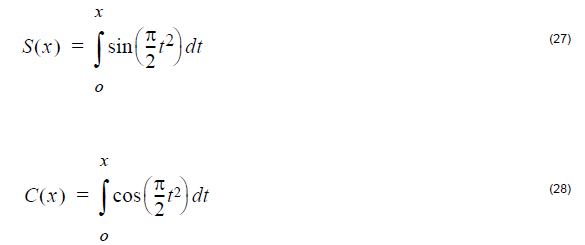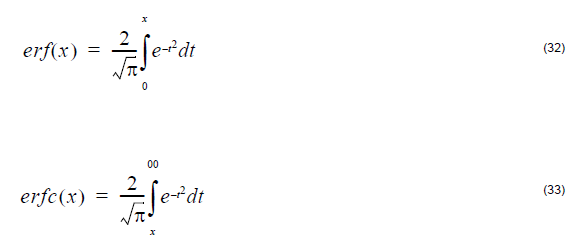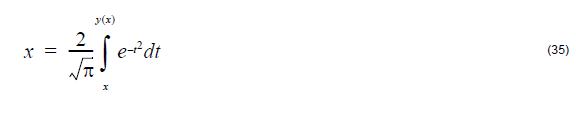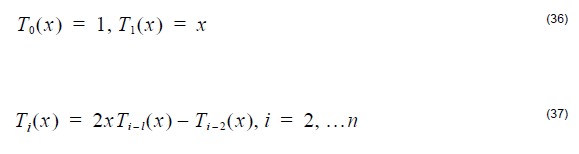## Overview of Script Language

The script language OptiFiber uses is a programming language based on the BASIC
language syntax. You use the script language to define the fiber profile, or material
dispersion, for example. To start programming with the Script Language, you have to
choose the User Defined option in OptiFiber dialog boxes.

The script language is described in the following reference sections:

• Variables, Arrays, and Operators: Variables, Arrays, Mathematical Operators,
Boolean Operators, and Comparison Operators
• Commands and Statements: Commands and Statements, RETURN Command,
IF Statement, ERROR Keyword, FIRSTTIME Keyword, Input Dialog Box,
Example Input, and Example Ginput
• Predefined Constants: Mathematical Constants and Physical Constants
• Functions Library: Commonly Used Functions, Other Functions, Fresnel Integral,
Gamma Function, Error Function, Bessel Functions, Chebyshev Polynomials
• Unit Conversions: Length Conversions, Temperature Conversions, Other
Conversions

## Variables, Arrays, and Operators

### Variables

A variable name can be any string beginning with a character. You cannot use special
characters and operators in the name. Some names are reserved for commands,
predefined constants, and functions. Variable names are case sensitive. For
example, ‘a’ is not the same as ‘A’, ‘Pos’ is not the same as ‘pos’ or ‘POS’.

Examples:

Valid variable names: a, pos, lambda, width1

Invalid variable names: *a, _pi, IF, RETURN

Note: Comment lines in the editing area are preceded by the double slash symbol
//.

### Arrays

You can use only one-dimensional arrays. You do not have to declare an array before
using it. For the array index, you can use a positive or a negative integer. The usage
format is array_name[index].

Examples:
A
B[-3]
a[x]=x*2

### Mathematical Operators

The script language supports the following standard mathematical operators (listed in
decreasing priority):

^ power operator
* multiplication operator
/ division operator
– subtraction operator

### Boolean operators

The script language supports the following standard Boolean operators:

= ‘equal to’ operator
< ‘less than’ operator
> ‘more than’ operator
& ‘and’ operator
| or’ operator
Examples:
A=b
A<b
B&c
C|d

### Comparison operators

Comparison operators serve to establish relations between two objects. They can be
combined, for example:

a<=b; a<>b; a=>b

## Commands and Statements

### Commands and statements

The script language has a set of commands and control statements. All command and
statement names must be in upper case letters.

Examples:

IF is a command
If or if can be a variable, but is not a command

### RETURN command

The RETURN keyword terminates execution of the program in which it appears and
returns the control (and the value of expression, if given). Notice that if you don’t
specify RETURN in your program, the interpreter will return the value of expression
in the last logical order. You usually use the RETURN command if your program uses
IF – THEN commands.

Syntax: RETURN[expression]

Example 1:

A program with only one line (you don’t have to specify RETURN):

sin(3)*24+13*(2+6)

returns 107.38688.

The same result is obtained from the program:

RETURN sin(3)*24+13*(2+6)

Example 2:

Consider the program:

cp = 100
a = Length-x
IF x<cp THEN
RETURN x
ELSE
RETURN a

The above program returns x if x is less than 100. Otherwise, it returns Length-x if x
is larger or equal than 100.

Example 3:

Let’s modify the previous example, Example 2, as follows:

cp = 100
a = Length-x
IF x<cp THEN
b = x
ELSE
b = a
RETURN b

This example gives the same result as Example 2. The difference is that another
variable b is used instead of returning the value of a directly.

### IF statement

The IF statement controls conditional branching. The body of an IF statement is
executed if the value of the expression is nonzero. The syntax for the IF statement
has two forms.

Syntax: IF expression THEN statement ELSE statement

In both forms of the IF statement, the expressions, which can have any value, are
evaluated, including all side effects.

In the first form of the syntax, if expression is nonzero (true), the statement is
executed. If expression is false, statement is ignored. In the second form of syntax,
which uses ELSE, the second statement is executed if expression is false. With both
forms, control then passes from the If statement to the next statement in the program.

Examples:

The following are examples of the IF statement:

IF i > 0 THEN y = x / i ELSE x = i

In this example, the statement y = x/i is executed if i is greater than 0. If i is less than
or equal to 0, i is assigned to x.

For nesting IF statements and ELSE clauses, use BEGIN – END to group the
statements and clauses into compound statements that clarify your intent. If no
BEGIN – END are present, the interpreter resolves ambiguities by associating each
ELSE with the closest IF that lacks an ELSE.

IF i > 0 THEN // Without BEGIN – END
IF j > i THEN
x = j
ELSE
x = i

The ELSE clause is associated with the inner IF statement in this example. If i is less
than or equal to 0, no value is assigned to x.

IF i > 0 THEN
BEGIN /* With BEGIN – END */
IF j > i THEN
x = j
END
ELSE
x = i

The BEGIN – END enclosing the inner IF statement in this example makes the ELSE
clause part of the outer IF statement. If i is less than or equal to 0, i is assigned to x.

### ERROR keyword

The ERROR keyword stands for a constant and returns the error code (if any). In case
of a mathematical error, like division by zero or overflow, it will reset the error flag.

The constant returns the error code (if any) and in cases of mathematical errors
(division by zero, overflow, etc.) resets the error flag. This allows you to detect any
unexpected errors during calculation. The best example is to use the ERROR
command in the IF-THEN-ELSE statement.

Example 1:

The following one-line program:

Length /x

results in the Error message: Division by zero, b=Length/x?????

This is, because initially x has been set to 0.

Example 2:

Consider the program:

b=Length/x
IF ERROR THEN
b=100;
RETURN b

Now, whenever we have a mathematical error, b is set to 100 and the program will
continue without the error message.

Note: ERROR is a constant, and you cannot assign any other value to it.

### FIRSTTIME keyword

The FIRSTTIME keyword is a flag that indicates the first time internal run.

Example:

S =7
IF FIRSTTIME THEN
RETURN 100
RETURN x*s

### Input dialog box

This dialog will appear if you use INPUT or GINPUT in User Defined Functions. I t
contains a single edit box.

Syntax:

INPUT variable
INPUT “string” variable
INPUT “string” variable = init value

GINPUT variable=init value
GINPUT “string” variable = init value

### Example Input

Usage of INPUT is limited. Because in OptiFiber you use user defined functions
usually in a loop, INPUT will ask you to input data every time you repeat the loop
(usually more than 100 times). To avoid this and to avoid redundant programming (IF
FIRSTTIME THEN …), use the function GINPUT (general input), which will ask you
for input data only the first time when you enter the loop.

INPUT variable
INPUT “string” variable
INPUT “string” variable = init value

Example:

b=1
MAX = 8
INPUT “Factorial of:” MAX

You will get the same effect if you use:

b=1
INPUT “Factorial of:” MAX = 8

You will be asked to type a value in the Fractional Of box in the Input window.

Note: The initialization of MAX before INPUT is not necessary. If you delete the
line MAX = 8, the default value in the Input box will be 0.

### Example Ginput

GINPUT variable=init value
GINPUT “string” variable = init value

(general input)

Displays an Input window on the screen (text is optional).

If the whole program (User Defined Function) is running more than once in the logical
(internal) block,

GINPUT will ask you to enter data only the first time the program runs.

Example:

If you define the following user function:

s=4
tanh(s*(x/Length))*(tanh (s*(1-x/Length)))

then the shape of the above function is controlled by the s parameter (assuming that
x is a running variable).

Now, if you want to test different shapes, with different s values, you will need to
change the s value for each calculation. Instead of going through all dialog boxes and
buttons to reach the User Function dialog box, whenever you want to change “s”.

Here comes the advantage of the GINPUT. You can use the GINPUT function:

GINPUT “Input Shape parameter” s=4
tanh(s*(x/Length))*(tanh (s*(1-x/Length)))

You will be asked to input different “s” values each time you press the Calculation
button. Thus, you will be able to try different function shapes very quickly and easily.

## Predefined Constants

### Mathematical constants

pi 3.14159265358979323846

e 2.71828182845904523536

### Physical constants

 Symbol Value Units Meaning _c 2.9979e8 m/s Speed of light in free space _e 8.8542e-12 F/m Permittivity in free space _mi 4*pi*10e-7 H/m Permeability in free space _q 1.60219e-19 C Elementary charge _me 9.1095e-31 kg Free electron mass _u 1.660531e-27 kg Atomic mass unit _mp 1.672614e-27 kg Proton rest mass _mn 1.674920e-27 kg Neutron rest mass _eV 1.60219e-19 J Energy unit (electron-volt) _h 6.626e-34 J s Planck constant _hr 1.05459 e-34 Js Reduced Planck Constant _lc 2.4263096e-12 m Compton wavelength of electron _Ry 13.6058 eV Rydberg energy _ri 1.09737312e7 1/m Rydberg constant _kT 25.853 meV Thermal energy _NA 6.022045e23 1 Avogadro number _f 9.648 6e4 C/mol Faraday constant _a 7.297351e-3 1 Fine structure constant _a0 5.291771e-11 m Bohr radius _re 2.817939e-15 m Electron radius _mb 9.274096e-24 J/T Bohr magnetron _kB 1.3807e-23 J/K Boltzmann constant _sb 5.66961e-8 1 Stefan-Boltzmann constant

## Functions Library

### Other functions

min(a,b) =a if a<b else b
max(a,b) =a if a>b else b
comb(n, k)= number of combinations for k object, n – total number of objects (n>=m),
return 0 if error
perm(m, n) =permutation mPn (0 if error)
gcd(a, b) = Greatest Common Divisor between a & b
lcm(a, b) = Largest Common Multiple between a & b
frc(x) = fractional part of ‘x’
int(x) = integer part of ‘x’
angle(x,y) Computes angle from Cartesian position (x,y), angle defined positive if y
>0 and negative if y <0
rand(MaxNum) => Randomize {0..MaxNum}

### Fresnel integral

fresnel_s(x) = Fresnel Integral SIN
fresnel_c(x) = Fresnel Integral COS

Evaluates the Fresnel integralsf(x), g(x)
fresnel_f(x) = function f(x) related to the Fresnel Integral
fresnel_g(x) = function g(x) related to the Fresnel Integral

Evaluates the functions f(x) and g(x) related to the Fresnel integrals by means of the
formula

### Computes the value of the gamma function at x

lgamma(x) Natural logarithm of Gamma Function, x – must be positive

### Error function

erf(x) error function

erfc(x) complementary error function

Computes the error function erf(x) and Complementary error function erfc(x):When x>26 then erf(x)=1 and erfc(x)=0

When x< -5.5 then erf(x)=-1 and erfc(x)=2;

nexperfc computes exp(x2)∗erfc(x)

Inverse error function y(x):

inverf(x,minx)

Evaluates the inverse error function y(x) wherex – it is necessary that -1 <x < 1

if |x| > 0.8 then value of x is not used in the procedure

minx if |x| <= 0.8 then value minx is not used in the procedure

if |x|> 0.8 then minx has to contain the value of 1-|x|.

In the case that |x| is in the neighborhood of 1, cancellation of digits take place in the
calculation of 1-|x|

If the value 1-|x| is known exactly from another source, then minx has to contain this
value, which will give better results.

### Bessel functions

#### Bessel functions J

bessj0(x) – Bessel function J0(x) of the 1st kind of order 0

bessj1(x) – Bessel function J1(x) of the 1st kind of order 1

bessj [k] (x) – Bessel function Jk(x) of the 1st kind in order k

#### Bessel functions Y (Weber’s function )

bessy0(x) – Bessel function Y0(x) of the 2nd kind of order 0

bessy0(x) – Bessel function Y1(x) of the 2nd kind of order 1

bessy [k] (x) – Bessel function Yk(x) of the 2nd kind of order k

#### Modified Bessel functions I

bessi0(x) – Modified Bessel function I0(x) of the 1st kind of order 0

bessi1(x) – Modified Bessel function I1(x) of the 1st kind of order 1

bessi [k] (x) – Modified Bessel function Ik(x) of the 1st kind of order k

#### Modified Bessel functions K

bessk1(x) – Mod. Bessel function K0(x) of the third kind of order 0

bessk1(x) – Mod. Bessel function K1(x) of the third kind of order 1

bessk [k] (x) – Mod. Bessel function Kk(x) of the third kind of order k

#### Spherical Bessel functions

spbessj[k](x) => Spherical Bessel funct. Jk+0.5(x)

#### Bessel functions of the second kind (Neumann’s functions)

neumann(a,x) – Neumann function Ya(x) of the 2nd kind of order a

neumann1(a,x) – Neumann function Ya+1(x) of the 2nd kind of order a

#### Chebyshev polynomials

chepol(n,x) – Computes the value of the Chebyshev polynomial Tn(x)

##Unit Conversions

### Length conversions

ft_m(foot) foot to meter
yd_m(yard) yard to meter
in_m(inch) inches to meter

### Temperature conversions

c_k(celsius) Celsius to Kelvin
fh_k(Fahrenheit) Fahrenheit to Kelvin
fh_c(Fahrenheit) Fahrenheit to Celsius
k_c(kelvin) Kelvin to Celsius
c_fh(celsius) Celsius to Fahrenheit
k_fh(kelvin) Kelvin to Fahrenheit

### Other conversions

gal_l gal_l(gallon) gallon to liter
pt_l pt_l(pint) pint to liter
oz_l oz_l(oz) oz to liter
in3_l in3_l(cubInc) cubic inch to liter
l_m3 l_m3(liter) liter to cubic meter
pd_kg pd_kg(pound) pound to kilogram
phe phe(wavelength[ìm]) calculate Photon energy [eV]